Addition and Subtraction of Unlike Fractions

Chapter 7 Class 6 Fractions
Concept wise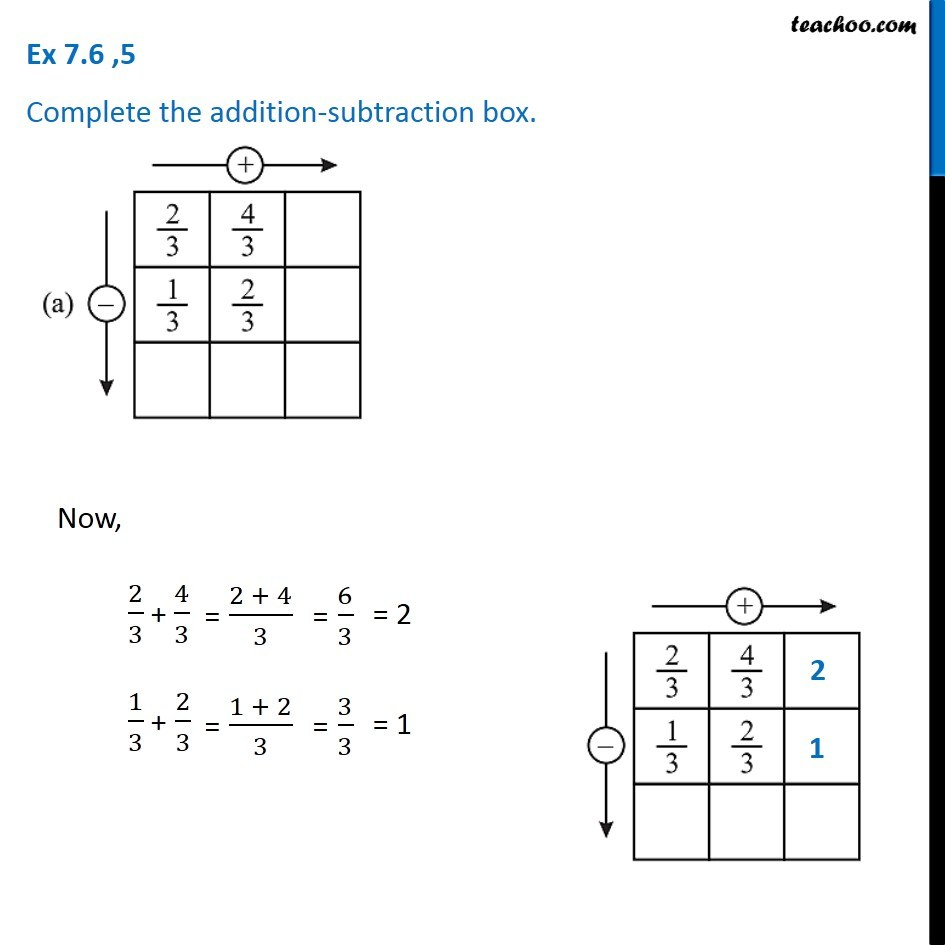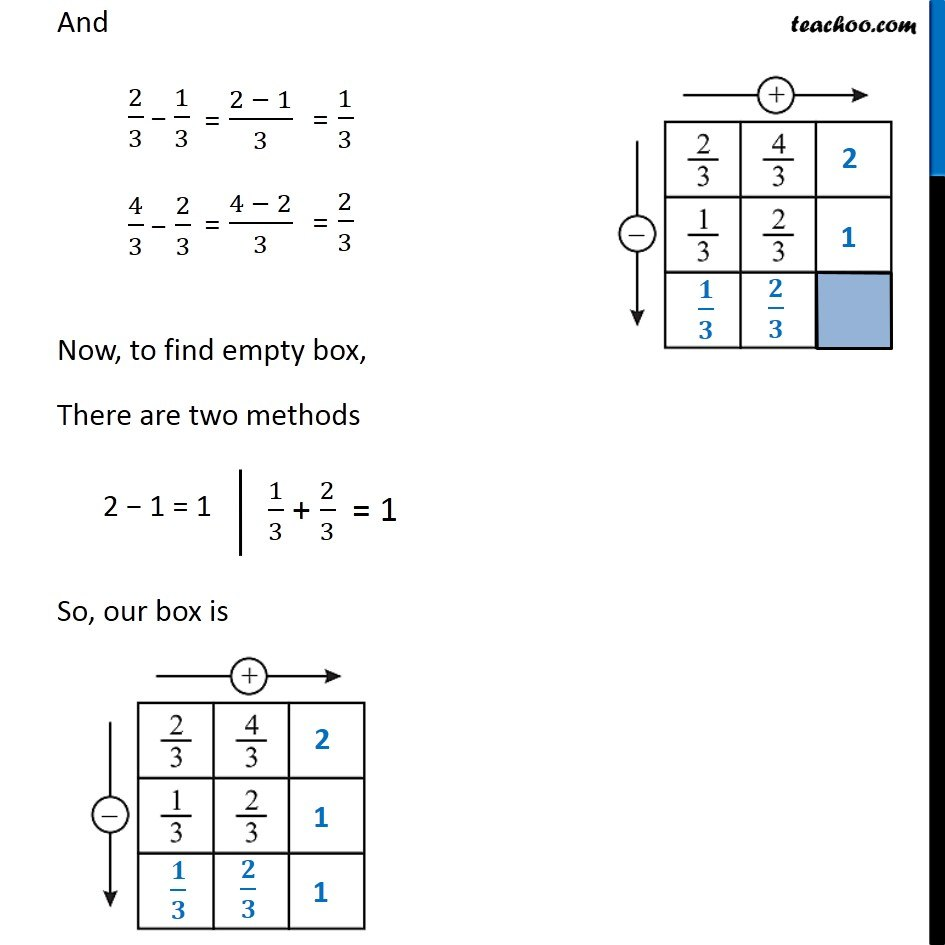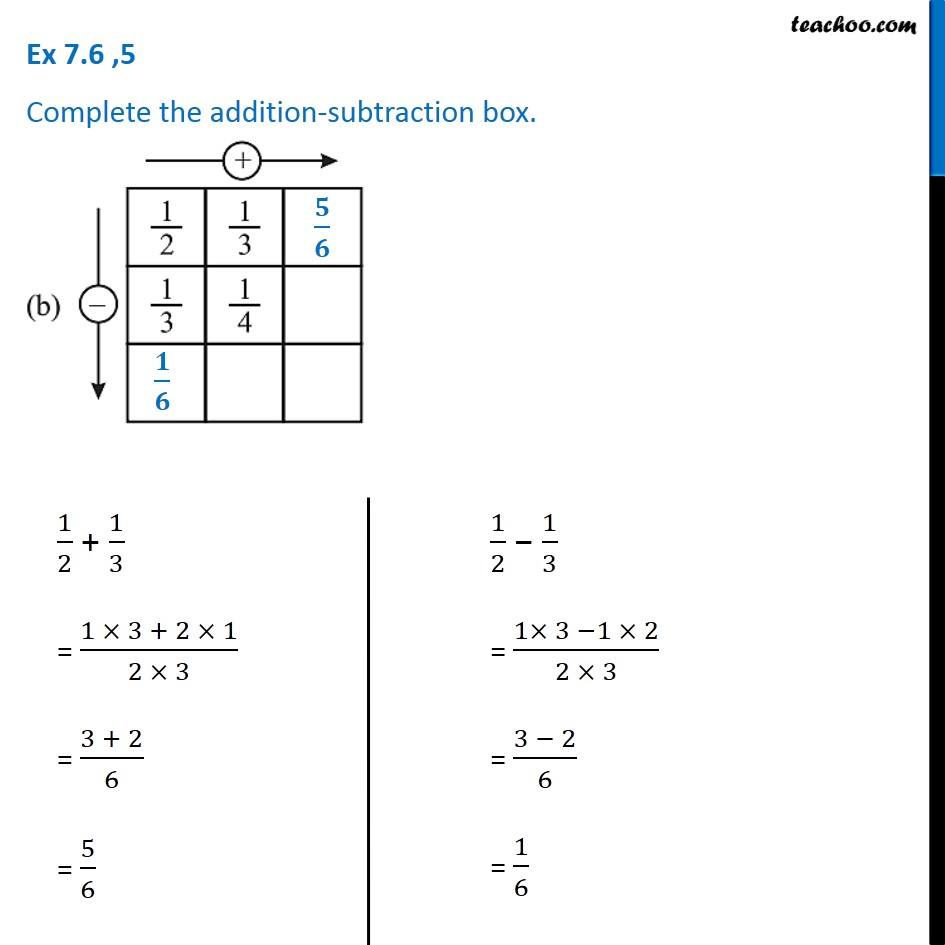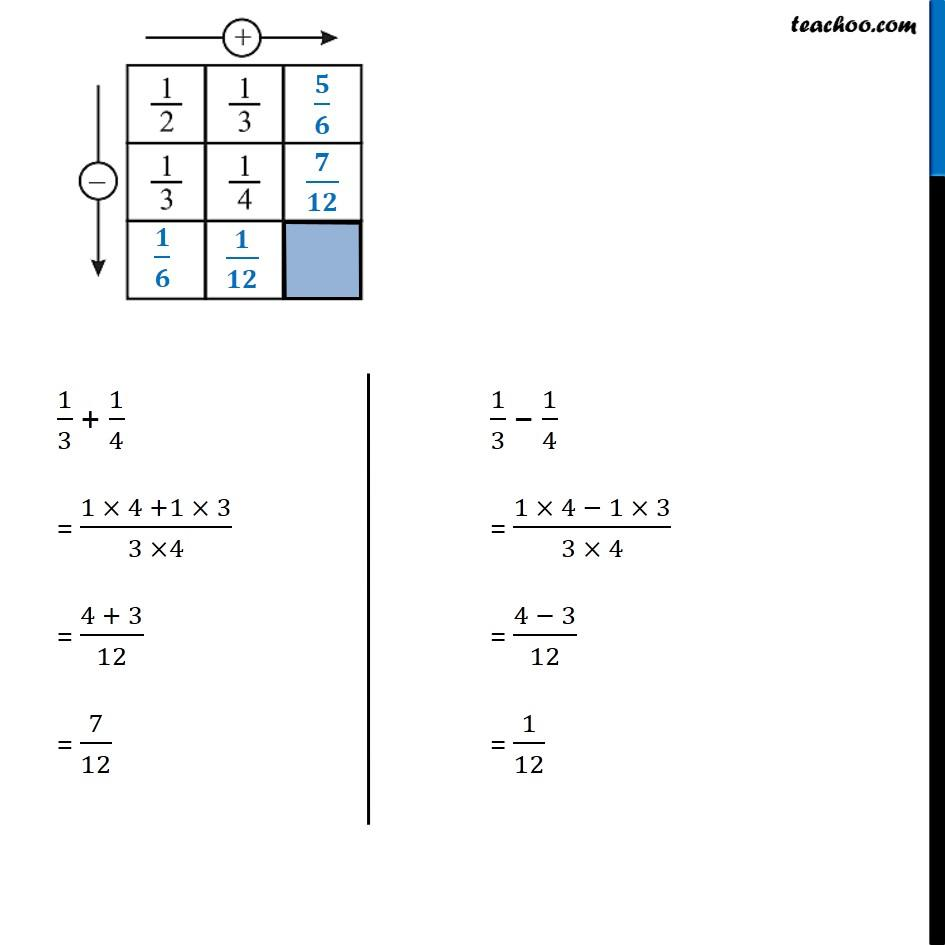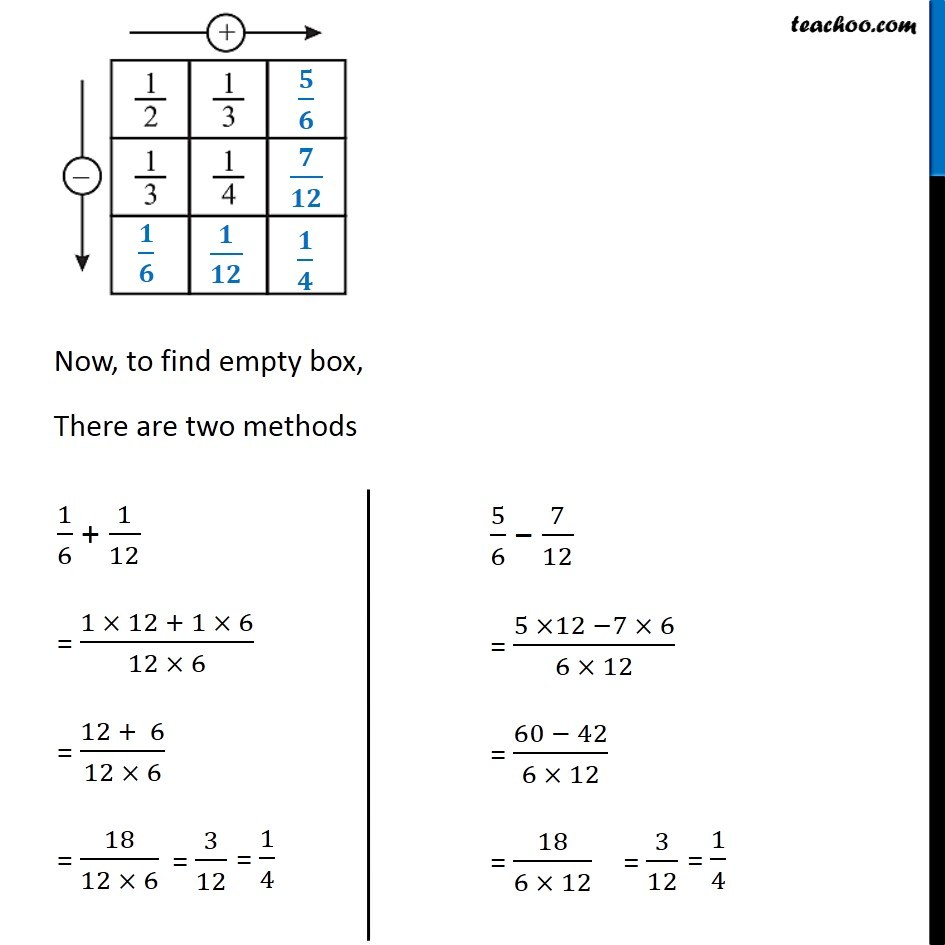### Transcript

Ex 7.6 ,5 Complete the addition-subtraction box.Now, 2/3 + 4/3 1/3 + 2/3 And 2/3 − 1/3 4/3 − 2/3 Now, to find empty box, There are two methods So, our box is Ex 7.6 ,5 Complete the addition-subtraction box.1/2 + 1/3 = (1 × 3 + 2 × 1)/(2 × 3) = (3 + 2)/6 = 5/6 1/2 − 1/3 = (1× 3 −1 × 2)/(2 × 3) = (3 − 2)/6 = 1/6 1/3 + 1/4 = (1 × 4 +1 × 3)/(3 ×4) = (4 + 3)/12 = 7/12 1/3 − 1/4 = (1 × 4 − 1 × 3)/(3 × 4) = (4 − 3)/12 = 1/12 Now, to find empty box, There are two methods 1/6 + 1/12 = (1 × 12 + 1 × 6)/(12 × 6) = (12 + 6)/(12 × 6) = 18/(12 × 6) 5/6 − 7/12 = (5 ×12 −7 × 6)/(6 × 12) = (60 − 42)/(6 × 12) = 18/(6 × 12)